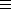## CGAL::Box_intersection_d::Box_d<NT,int D,IdPolicy>

### Definition

Box_intersection_d::Box_d<NT,int D,IdPolicy> is a generic iso-oriented bounding box in dimension D. It provides in each dimension an interval with lower and upper endpoints represented with the number type NT. This class is designed to work smoothly with the algorithms for intersecting sequences of iso-oriented boxes. For degeneracy handling, the boxes need to provide a unique id-number. The policy parameter IdPolicy offers several choices. The template parameters have to comply with the following requirements:

• NT: number type for the box boundaries, needs to be a model of the Assignable and the LessThanComparable concept.
• int D: the dimension of the box.
• IdPolicy: specifies how the id-number will be provided. Can be one of the following types, where ID_EXPLICIT is the default for this parameter:
• ID_NONE: no id-number is provided. Can be useful if Box_d is used as a base class for a different implementation of id-numbers than the ones provided here.
• ID_EXPLICIT: the id-number is stored explicitly in the box and automatically created and assigned at construction time of the box. Note that copying a box (copy-constructor and assignment) does not create a new id-number but keeps the old one, which is the behavior needed by the CGAL::box_self_intersection algorithm. This is therefore the safe default implementation.
• ID_FROM_BOX_ADDRESS: casts the address of the box into a std::ptrdiff_t to create the id-number. Works fine if the intersection algorithms work effectively with pointers to boxes, but not in the case where the algorithms work with box values, because the algorithms modify the order of the boxes, and the CGAL::box_self_intersection algorithm creates copies of the boxes that would not have identical id-numbers.

#include <CGAL/Box_intersection_d/Box_d.h>

and also automatically with
#include <CGAL/box_intersection_d.h>

### Types

 Box_intersection_d::Box_d::NT number type to represent the box boundaries. Allowed are the built-in types int, unsigned int, float, and double.

 typedef std::size_t ID; type for the box id-number.

### Creation

Box_intersection_d::Box_d<NT,int D,IdPolicy> box;
Default constructor. No particular initialization.

Box_intersection_d::Box_d<NT,int D,IdPolicy> box ( bool complete);
initializes to the complete or the empty space. If empty, all interval starting(end) points will be set to positive(negative) infinity.

Box_intersection_d::Box_d<NT,int D,IdPolicy> box ( NT lo[D], NT hi[D]);
initializes the box intervals to [lo[i],hi[i]], 0i < D.
 Precondition: lo[i] < hi[i] for 0i < D.

Box_intersection_d::Box_d<NT,int D,IdPolicy> box ( Bbox_2 bbox);
constructs from bbox
 Requirement: D=2 and NTdouble.

Box_intersection_d::Box_d<NT,int D,IdPolicy> box ( Bbox_3 bbox);
constructs from bbox
 Requirement: D=3 and NTdouble.

### Modifiers

 void box.init ( bool complete = false) initializes to the complete or the empty space. If empty, all interval starting(end) points will be set to positive(negative) infinity. void box.extend ( NT point[D]) extend box to contain the old box and point.

### Access Functions

int Box_d::dimension () returns D, the dimension of the box.

std::size_t box.id () returns a unique box id, see the IdPolicy template parameter above for the different choices.
 Requirement: IdPolicyID_NONE

NT box.min_coord ( int d) returns the lower boundary in dimension d
 Precondition: 0d < D

NT box.max_coord ( int d) returns the upper boundary in dimension d
 Precondition: 0d < D

Bbox_2 box.bbox () returns the bounding box
 Requirement: D=2 and NTdouble
Bbox_3 box.bbox () returns the bounding box
 Requirement: D=3 and NTdouble

void box.extend ( NT p[N]) extends box to the smallest box that additionally contains the point represented by coordinates in p.

void box.extend ( std::pair<NT,NT> p[N])
extends box to the smallest box that additionally contains the point represented by coordinate intervals in p.